﻿ Einstein mc^2 is wrong

# Einstein  mc2  is  wrong !

## Einstein  mc2  is  illusion.

### [ Mass increase depends on observer's movement ? ]

(Fig.1)  ↓ Mass (= energy ) change is "relative", NOT absolute value.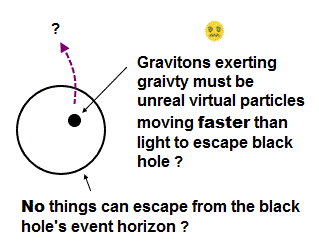Most textbooks claim that Einstein famous equation of E = mc2 contributes to nuclear power plant and atomic bomb.  But it's a total lie !

Because Einstein mass increase proportional to total energy has nothing to do with true energy.  It changes depending on observer !

Seen by stationary observer (= K in Fig.1 ), the upper object is moving at v and increases its total kinetic energy and mass.

But seen by moving observer (= K' ), the upper object appears to stop, so its relativistic mass and energy do NOT increase.

In Einstein relativity, there is NO absolute true energy ( and mass ), because they change depending on observer's motion.

So you cannot utilize these "illusory" energy (= mass ) in actual atomic power generation and bombs.

### [ Special relativity contradicts de Broglie theory. ]

(Fig.2)  Observer moves → interference disappears from screen ?The most fatal flaw in Einstein special relativity is that it contradicts de Broglie theory.

de Broglie theory says the electron wavelength depends on its velocity, and can be confirmed in various experiments such as double-slit.

Seen by stationary observer (= K ), the electron is moving at v and generates its de Broglie wave causing interference pattern on the screen.

But seen from moving observer (= K' ), the electron appears to stop, so it generates neither de Broglie wave nor interference on the screen !

This is clearly a paradox, and shows that Einstein relativity and mass are wrong.

## de Broglie wave disobeys Einstein.

### [ observer starts to move → de Broglie wave disappears !? ]

(Fig.3)  ↓ de Broglie wavelength (= λ ) contradicts Lorentz contraction.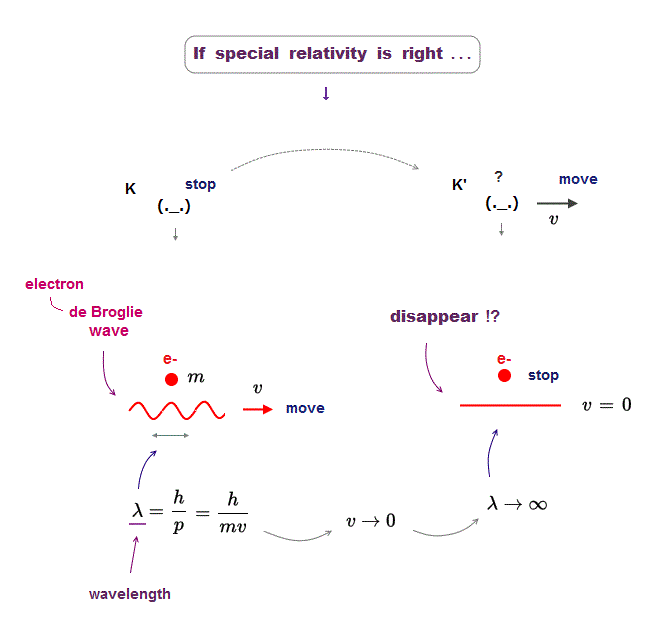The important point is that de Broglie wavelength disobeys Lorentz contraction (= special relativity ).

The moment an observer starts to move at the same speed as the electron, its de Broglie wavelength suddenly disappears.

This drastic change of electron's de Broglie wavelength contradicts mild change by Lorentz contraction in the same space.

As a result, de Broglie wave (= confirmed in experiments ! ) proves that Einstein relativity is wrong.

### [ Einstein claims force changes depending on observer. ]

(Fig.4)  Obsesrver moves (= K' ) → force Fy decreases ? → paradox !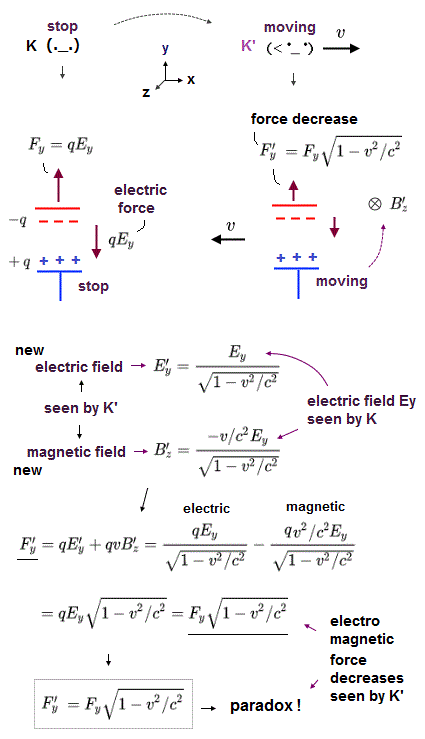Einstein energy (= mass ) and momentum relation has nothing to do with the original special relativity, because they contradict each other.

If you try to apply Einstein relativity to his relativistic momentum relation, you need to artificially change "force Fy", which causes paradox !

They claim that when the observer starts to move (= K' ), the force Fy applied to the object weakens ( this p.15,  this p.3 ).

## Two forces are balanced seen by K.

### [ The rod stops due to balanced upper and lower forces in K. ]

(Fig.5)  Seen by stationary observer K, the whole rod stops.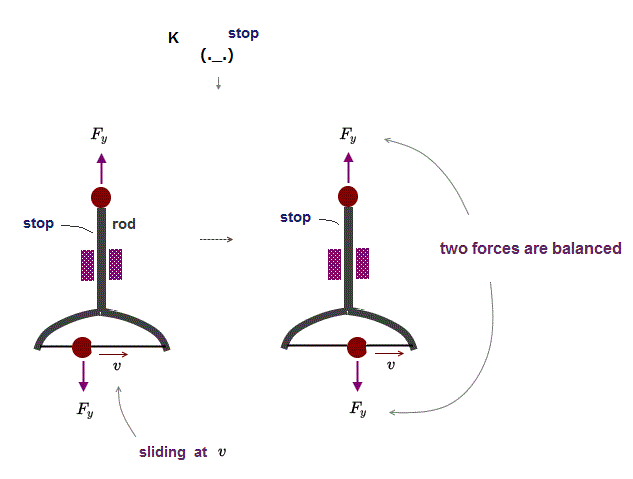In Fig.5, two forces Fy applied to the upper and lower parts of the rod are the same, so the whole rod stops under "balanced" forces.

On the lower part, the ball ( and the force applied to it ) connected to the rod is sliding at the velocity v.

Seen by stationary observer K, upper (= stop ) and lower (= sliding ) forces are balanced, and the whole rod stops.

## Observer moves → the rod moves downward ?

### [ Seen by moving observer K', two forces are unbalanced ! ]

(Fig.6)  The rod stops or moves downward depending on observer ?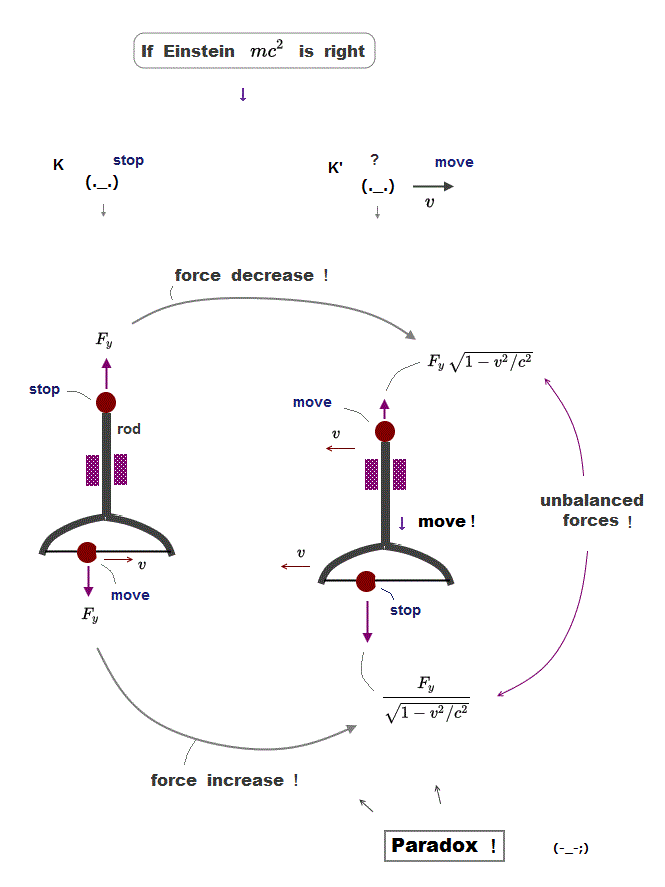Seen by stationary observer K, the whole rod stops, because the upper and lower forces are balanced.

But when the observer starts to move, the lower force increases, and the upper force decreases, and the rod starts to move downward !

This is clearly a paradox and proves Einstein mass energy relation is wrong.

It's unrealistic that whether the whole rod stops or moves downward depends on the observer motion ( K or K' ) !  Nonsense.

## Anti-matter doesn't exist.

### [ Rare anti-matter is just a "statistical artifact" of other particles. ]

(Fig.7)  Antimatter is too rare, only one in "millions" of proton collisions !?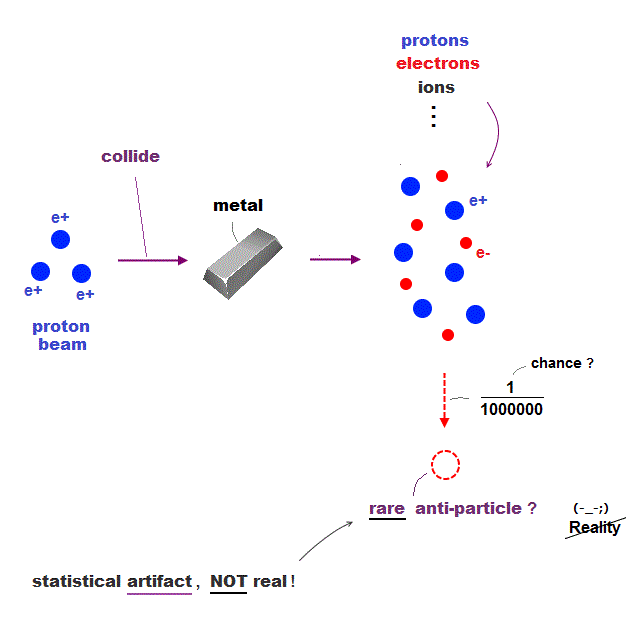Producing rare anti-matter depends on very disordered experiments where they claim millions of collisions between protons and metal generate only a few anti-protons.

In these infinite unrelated particles, some of them "look like" unreal anti-particles due to "multi-particle scattering" or something.

So spectrum of doubtful anti-hydrogen is just the result of their misinterpretation of spectrum from a pair of real proton and electron.

They just pick up only convenient fake data from a mixture of many kinds of unrelated high-energy particles and gamma rays using artificial simulation.

So as long as they cannot extract pure anti-matter only from pure light, anti-matter is just a statistical artifact of other unrelated particles.

## PET uses electron capture, NOT anti-particle !

### [ Positron emission = electron capture ?  ← NO antimatter ! ]

(Fig.8)  ↓Positron (= antimatter ) emission is impossible.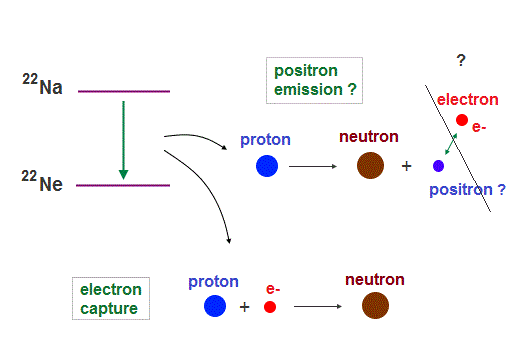They say antimatter is useful (← ? ) as PET in hospital.
The point is they do not detect antimatter but emitted electromagnetic wave.

In fact, "positron emission" in PET can be replaced by real "electron capture", because they both have the same effect on a nucleus ( this p.3 ) !

In Na nuclide, they argue both positron ( β+ ) emission and electron capture produce the same Ne.  ← Emitted "light" energy is the same, indistinguishable.

Positron emission is unrealistic, because a proton decays into a heavier neutron, causing "fantasy" perpetual machine !

So real "electron capture" is what actually happens in PET instead of unreal "positron emission".  = antimatter is useless.

## Einstein cannot explain true mechanism of mc2 !

### [ Potential energy decreases → total mass decreases, too ? ]

(Fig.9)  Electron & proton stop, but their total mass decreases !?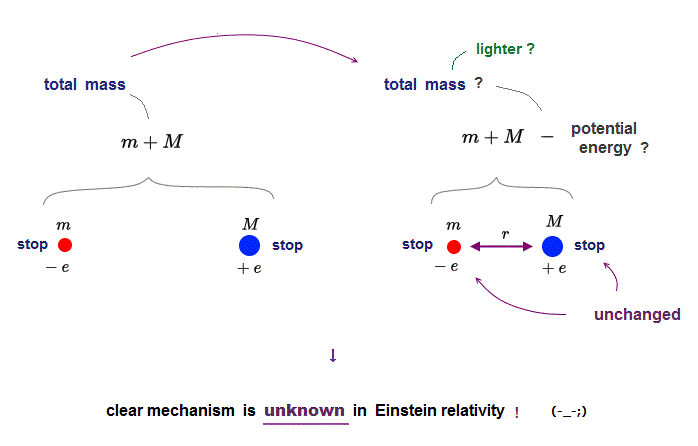Einstein relativity avoids true mechanism of why mass decrease is equal to total energy (= mc2 ) decrease.

In fact, this relation E = mc2 is very hard to confirm, because it's almost impossible to collect and measure all emitted energies such as unseen neutrino, γ rays, and new particles.

But as total energy decreases, the total mass appears to slightly decrease in actual experiments.

Einstein claims that even if an electron and a proton remain stopping, their total mass can decrease when Coulomb potential energy decreases.

This mechanism cannot be explained by Einstein original postulate, because the motion of electron and proton is unchanged in left and right in Fig.9.

## Medium is needed for mass increase.

### [ Particle mass is unchanged, but total mass increases = medium is needed. ]

(Fig.10)  Mass increase = increased resistance from surrounding medium.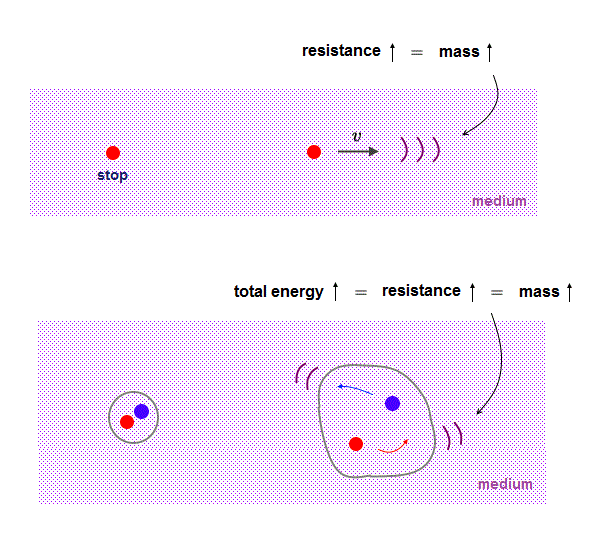Einstein claims that even when particle's original ( rest ) mass remains unchanged, its total mass appears to increase as it speeds up.

This relativistic explanation is contradictory.  If the original particle's mass remains the same, we need other material to explain its mass increase.

As a particle accelerates, the resistance from the surrounding medium becomes stronger, which is detected as "mass increase effect".

Because the maxium transmission speed in the medium is light speed c, each particle cannot exceed c due to increased resistance.

In the same way, as the total energy is higher, each particle is moving around more vigorously, which gives and receives more resistance from the surrounding medium.

Higher Coulomb potential energy means each particle feels more resistance from the surrounding medium than the lower potential energy places.

So without the medium around particles, the energy-mass relation can never be explained.2017/5/12 updated. Feel free to link to this site.